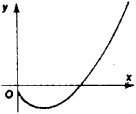# Natural Boundary Point

The following article is from The Great Soviet Encyclopedia (1979). It might be outdated or ideologically biased.

## Natural Boundary Point

a singular point of a curve at which the curve terminates. In Figure 1, for example, the origin is a natural boundary point for the curve y = x ln x, since for x < 0 the function In x is not defined for the real numbers.Figure 1

[20–1541–]